﻿ 多点地质统计学算法的C#实现及应用 Realization and Application of Multipoint Geostatistics Algorithms by C#

Software Engineering and Applications
Vol. 08  No. 02 ( 2019 ), Article ID: 29710 , 7 pages
10.12677/SEA.2019.82007

Realization and Application of Multipoint Geostatistics Algorithms by C#

Fengyun Guo, Changyun Miao

The 22nd Research Institute of CETC, Xinxiang Henan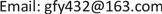Received: Mar. 24th, 2019; accepted: Apr. 8th, 2019; published: Apr. 15th, 2019ABSTRACT

In Snesim algorithm of multi-point geological statistics, the probability distribution can be determined by scanning the number of data events which are same as the distribution of conditional data in the training image, which can reflect the joint variability of multiple locations. In this paper, Snesim algorithm is implemented in C# language. Using the parallel operation ability of C# to build a search tree greatly improves the computational efficiency. The search algorithm of data events is studied in this paper, and the effect of changing training image to verify the treatment of simulation results is explored.

Keywords:Training Image, Search Tree, Data Events, Parallel ComputationCopyright © 2019 by authors and Hans Publishers Inc.1. 引言

2. 多点地质统计学基础

$P\left\{{d}_{n}\right\}=P\left\{S\left({u}_{\alpha }\right)={s}_{{k}_{\alpha }};\alpha =1,2,\cdots ,n\right\}\approx \frac{C\left({d}_{n}\right)}{{N}_{n}}$ (1)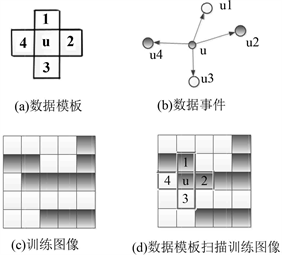Figure 1. Basic elements of multipoint geostatistics

$\text{Prob}\left\{S\left(u\right)={S}_{k}|{d}_{n}\right\}=\frac{\text{Prob}\left\{S\left(u\right)={S}_{k}\text{\hspace{0.17em}}and\text{\hspace{0.17em}}S\left({u}_{\alpha }\right);\alpha =1,2,\cdots ,n\right\}}{\text{Prob}\left\{S\left(u\right)={S}_{{k}_{\alpha }};\alpha =1,2,\cdots ,n\right\}}$ (2)

$P\left\{S\left(u\right)={S}_{k}|{d}_{n}\right\}\approx \frac{{C}_{k}\left({d}_{n}\right)}{C\left({d}_{n}\right)}$ (3)

3. 多点地质统计学随机建模方法及C#编程实现

3.1. Snesim算法

① 建立训练图像，选定训练图像后，按定义的坐标规则输入训练图像数据，本文定义的坐标原点为训练图像矩阵的左下角；

② 准备待模拟数据，将实测的井数据作为已知的条件数据标注在最近的网格结点上；

③ 用自定义的数据模板  扫描训练图像，以构建搜索树。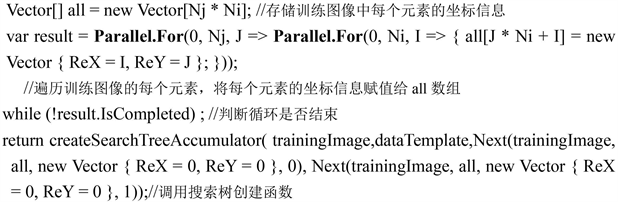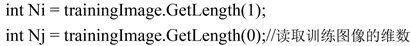Paraller. For()方法类似于C#的for循环语句，多次执行一个任务。使用Paraller. For()方法并行运行迭代，迭代的顺序没有定义。Paraller. For()方法的返回类型是ParallelLoopResult结构，它提供了循环是否结束的信息和最低迭代的索引。

④ 确定一个访问未取样结点的随机路径，从搜索树里提取多点概率分布，计算局部条件概率，建立未取样点局部条件概率分布；

⑤ 用蒙特卡洛方法  ，根据随机数的值分布区间决定待模拟点的属性值u。u的取值基于先验定义该区域的属性状态集合(本文定义两种状态0和1)，根据随机数的概率分布决定u取0或者1，并将实现值加入到条件数据中；

⑥ 重复步骤④、⑤，如果需要多个实现，只需改变随机访问路径，重复步骤④、⑤、⑥即可；

⑦ 改变随机路径，产生另一个随机模拟实现。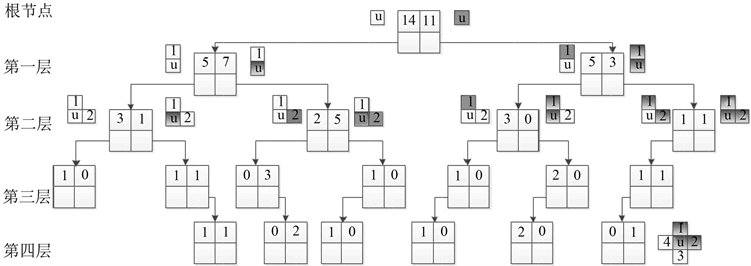Figure 2. Search tree structure

3.2. 搜索树中数据事件查找及条件概率提取算法

① 假如待模拟点u的条件数据u1、u2、u3、u4均不存在，则返回搜索树根节点；

② 假如待模拟点u的条件数据u1、u2、u3、u4均存在，还应判断条件数据的状态值是否可以在搜索树中找到相应的节点，若条件数据带u1、u2、u3、u4的数据事件在搜索树节点中不存在，则需依次减少条件数据的个数，直至找到去除所有的条件数据返回搜索树的根节点；

③ 假如待模拟点u的条件数据u1、u2、u3、u4不完全存在，则不存在的条件数据分两种状态(本文暂定两种状态0和1)与存在的条件数据状态组合成完全的条件数据，然后分两个分支判断节点是否存在，若存在节点，返回该节点中，若不存在则依次去掉较远的条件数据再去查找节点；两个分支取较少层数的长度(节点短的分支长度)，然后取相同层数的节点中的0和1状态的事件重复数对应相加，最终得到待模拟点为不同岩性状态的条件概率分布。

4. 数据仿真及实际地层岩性模拟应用

① 读入训练图像数据(见图3)，并定义一定的坐标系统对其进行存储。Figure 3. Training image data

② 将待模拟数据矩阵按与训练图像同样的坐标系统输入，并对待模拟矩阵进行一系列预处理，如矩阵边界的处理、待模拟点数据及其坐标的提取。

③ 确定数据模板的样式，本文采用的是图1(a)所示模板，数据模板确定后，便可构造搜索树，然后对训练图像进行扫描、数据事件的查找及待模拟点条件概率分布函数的生成等。

④ 按蒙特卡洛方法生成随机数决定待模拟点的岩性状态，替代原始待模拟数据矩阵中的未知状态−1。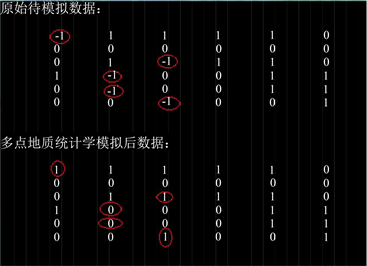Figure 4. The comparison of the raw data with the data after multi-point statistical simulation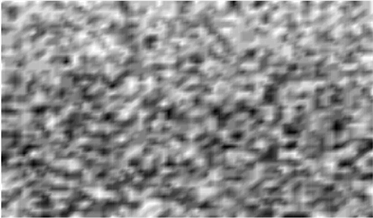Figure 5. The simulated result graph using training image T1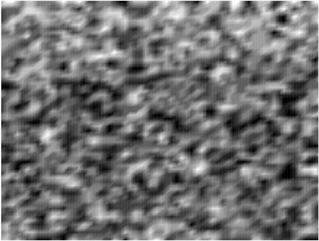Figure 6. The simulated result graph using training image T2

5. 结论

Realization and Application of Multipoint Geostatistics Algorithms by C#[J]. 软件工程与应用, 2019, 08(02): 58-64. https://doi.org/10.12677/SEA.2019.82007

1. 1. 李少华, 张昌民, 尹艳树. 储层建模算法剖析[M]. 北京: 石油工业出版社, 2012: 139-142.

2. 2. Strebelle, S. and Journel, A. (2001) Reservoir Modeling Using Multiple-Point Statistics. SPE Annual Technical Conference and Exhibition, New Orleans, Louisiana, 30 September-3 October 2001, SPE 71324. https://doi.org/10.2118/71324-MS

3. 3. 王芳芳, 陈小宏, 李景叶. 多点地质统计学整合地震数据的研究算法[C]//石油地球物理勘探物探技术研讨会专刊, 2012: 219-222.

4. 4. 高璐, 马玉志. 浅谈Microsoft Visual Studio 2010新特性[J]. 黑龙江科技信息, 2010(32): 96.

5. 5. 邢陈思. 探索Visual Studio 2010对并行计算的支持[J]. 金融科技时代, 2012, 20(1): 56-58.

6. 6. 张挺. 基于多点地质统计的多孔介质重构方法及实现[D]: [博士学位论文]. 合肥: 中国科学技术大学, 2009: 36-37.

7. 7. 熊哲, 严锡, 金杨波. 油藏地质建模中多点训练图像应用研究[J]. 江汉石油职工大学学报, 2012, 25(4): 1-2.